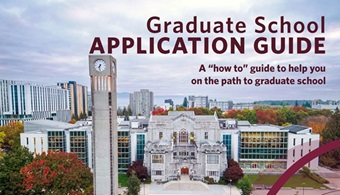# Richard Anstee

Professor

#### Affiliations to Research Centres, Institutes & Clusters

##### Doctoral Student Supervision

Dissertations completed in 2010 or later are listed below. Please note that there is a 6-12 month delay to add the latest dissertations.

Forbidden configurations (2011)

In this work we explore the field of Forbidden Configurations, a problem in Extremal Set Theory. We consider a family of subsets of {1,2,...,m} as the corresponding {0,1}-incidence matrix. For {0,1}-matrices F, A, we say F is a *subconfiguration* of A if A has a submatrix which is a row and column permutation of F. We say a {0,1}-matrix is *simple* if it has no repeated columns. Let ||A|| denote the number of columns of A. A {0,1}-matrix F with row and column order stripped is a *configuration*. Given m and a family of configurations G, our main function of study is forb(m,G) := max{||A|| : A simple and for all F in G, we have F not a subconfiguration of A }. We give a general introduction to the main ideas and previous work done in the topic. We develop a new more computational approach that allows us to tackle larger problems. Then we present an array of new results, many of which were solved in part thanks to the new computational approach.

View record

##### Master's Student Supervision

Theses completed in 2010 or later are listed below. Please note that there is a 6-12 month delay to add the latest theses.

On rigidity of unit-bar frameworks (2019)

A framework in Euclidean space consists of a set of points calledjoints, and line segments connecting pairs of joints called bars. Aframework is flexible if there exists a continuous motion of its jointssuch that all pairs of joints with a bar remain at a constant distance, but between at least one pair of joints not joined by a bar, the distance changes. Forexample, a square in the plane is not rigid since it can be deformedinto a family of rhombi. This thesis is mainly concerned with infinitesimal motions. Loosely speaking, a framework is infinitesimally rigid if it does not wobble. One example is a motion of a single joint, where all other joints are unmoving, such that the movement of the one joint is perpendicular to all bars attached to it. The distances in an infinitesimal motion are preserved in the initial instant of motion. Infinitesimally rigidframeworks are rigid, and is an easier quality to verify, thereby making it a popular notion of rigidity to study among engineerings, architects, and mathematicians. We present infinitesimally rigid bipartiteunit-bar frameworks in ℝ^n, and infinitesimally rigidbipartite frameworks in the plane with girth up to 12. Our constructions make use of the knight's graph; a graph such that vertices (joints) are squares of a chessboard and edges (bars) represent legal moves of the knight. We show that copies of the knight's graph can be assembled to create infinitesimally rigid frameworks in any dimension. Our constructions answer questions of Hiroshi Maehara.

View record

Families of forbidden configurations (2013)

The forbidden configuration problem arises from a question in extremal set theory. The question seeks a bound on the maximum number of subsets of an m-set given that some trace is forbidden. In terms of hypergraphs, we seek the maximum number of edges on a simple hypergraph of m vertices such that this hypergraph does not contain a forbidden sub-hypergraph. We will use the notation of matrices to describe the problem as follows. We call a (0,1)-matrix simple if it has no repeated columns; this will be the analogue of a simple hypergraph. Let F be a given matrix. We say that a (0,1)-matrix A contains F as a configuration if there is some submatrix of A that is a row and column permutation of F. This is equivalent to a hypergraph containing some sub-hypergraph. F need not be simple. We define forb(m, F ) as the maximum number of columns possible for a simple, m-rowed, (0,1)-matrix that does not contain F as a configuration. A variation of the forbidden configuration problem forbids a family of configurations F = {F₁, F₂, ... Fn} instead of a single configuration F. Thus, forb(m, F) becomes the maximum number of columns possible for a simple, m-rowed, (0,1)-matrix that does not contain any one of the matrices in the family as a configuration. We will present a series of results organized by the character of forb(m, F). These include a classification of families such that forb(m, F) is a constant and the unexpected result that for a certain family, forb(m, F) is on the order of m³/², where previous results typically had forb(m, F) on the order of integer powers of m. We will conclude with a case study of families of forbidden configurations and suggestions for future work.

View record

## Current Students & Alumni

This is a small sample of students and/or alumni that have been supervised by this researcher. It is not meant as a comprehensive list.

#### Membership Status

Member of G+PS
View explanation of statuses

#### Department(s)

If this is your researcher profile you can log in to the Faculty & Staff portal to update your details and provide recruitment preferences.